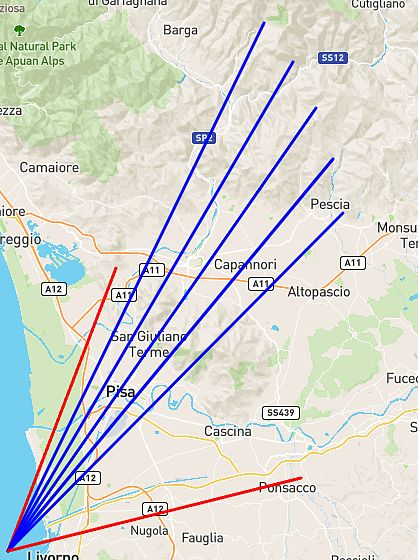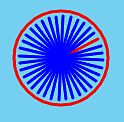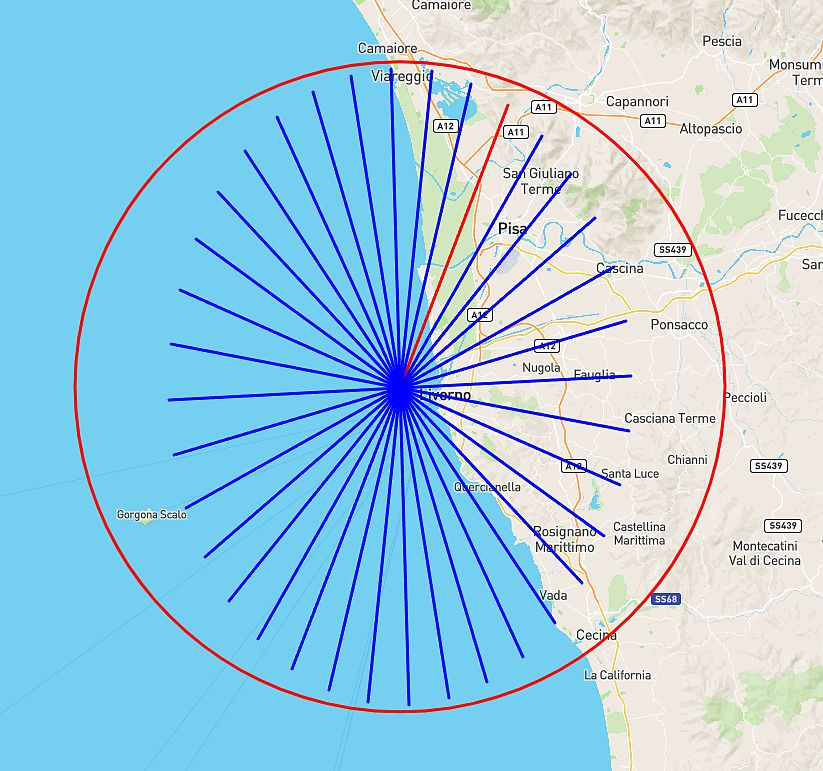# Leaflet and Math - Rotation of point in a Map

Hello everybody,
I write in the XoJo forum because I cannot find a solution to my problem on the Intenet and I hope there is someone who is familiar with Leaflet (link: Leaflet - a JavaScript library for interactive maps - https: // leafletjs .com).

I have developed an air navigation program with XoJo. I am almost at the end of the program and I would not like to give up right now for a nonsense to which I cannot find a solution.

The question I am about to ask you is not inherent to XoJo: it is about the representation of a rotated line in the Leaflet map.As you can see in the figure, there are two red lines C-> P1 and C-> P2 and there are blue lines C-> A, C-> B, …, C-> E.
The red line C-> P1 is the starting line, the C-P2 line is the finish line. They are input data.
The blue lines are lines I calculated with the following mathematical formula:

``````// Data input
Cx = 10.247500 '=> C
Cy = 43.557778 '=> C
Ax = 10.395555 '=> P1x
Ay = 43.838056 '=> P1y
Bx = 10.64953 '=> P2x
By = 43.62996 '=> P3y

// Calculate the angle of C-> P2 with the axis
Var z, w, Angle As Double
w = ((Ax-Cx) * (Bx-Cx)) + ((Ay-Cy) * (By-Cy))
z = Sqrt (((Ax-Cx) ^ 2) + ((Ay-Cy) ^ 2)) * Sqrt (((Bx-Cx) ^ 2) + ((By-Cy) ^ 2))
Angle = (ACos (w / z)) * 180 / Pi

Var iStepRotazione As UInteger = 10 'Let's start with 10° of rotation
Var xPoint, yPoint As Double
While iStepRotation <Angle
xPoint = (Ax-Cx) * Cos (iStepRotazione * Pi / 180) + (Ay-Cy) * Sin (iStepRotazione * Pi / 180) + Cx
yPoint = - (Ax-Cx) * Sin (iStepRotation * Pi / 180) + (Ay-Cy) * Cos (iStepRotation * Pi / 180) + Cy
iStepRotazione = iStepRotazione + 10 'Step of 10 °
sReturn = sReturn + Str (yPoint) + "," + Str (xPoint) + ";"
Wend
``````

That is, I take point P1 and rotate it by 10 ° until reaching P2.
With the calculated points, I create the lines (in Leaflet) C->A, C->B, etc …

As you can see in the figure, the rotated points (A, B, …, F) form smaller lines (the blue lines).

I’m not a great mathematician but I believe the function is correct.
Having said that, do you think it is a mathematical problem or a Web-Mercator problem?

Do you know anything about it?

Thank you.

This looks like an issue of the Mercator projection. I’ll need to get on a proper computer to be sure, but it looks like those lines are the same actual length on the globe, but appear to shrink when projected.

They seem to get smaller each time.
Im curious to know what happens if you go fully ‘round the clock’ ?

Can you confirm that ALL your variables are all doubles? (the dim / var lines are not shown here)

Are you sure that this code is current?

you could rotate by

xEnd = xStart + Cos(Radian) * distance
yEnd = yStart + Sin(Radian) * distance

(Start is C)

this cos/sin is more or less a direction, values from -1 to 1.
you go along with a multiplication.

To help me better, here you find the XoJo source and the HTML file to be able to see what XoJo calculates.

Hi MarkusR

``````Var Radian As Double = iStepRotazione * PI / 180 ' PI = 3.14159265
xPoint = Ax + Cos(Radian) * ??distance??
yPoint = Ay + Sin(Radian) * ??distance??
``````

Is the distance the length of the segment to be calculated (the blue line) ?

yes length is
dx=x2-x1
dy=y2-y1
length=sqrt(dx * dx + dy * dy)

C to P1

Markus,
your suggestion doesn’t work well (see image below). Thanks for helping me out anyway!

@J_Andrew_Lipscomb and @Jeff_Tullin also think it’s Web Mercator fault.
Let’s wait if they can give me a suggestion …Here is part of the code modified with your function:

``````//******* I rotate point P1 many times (10°) until I reach point P2
Var iStepRotazione As UInt16 = 10 'Step 10° rotation
Var dx, dy, length As Double
dx=Ax-Cx
dy=Ay-Cy
length=sqrt(dx * dx + dy * dy)
Var xPoint,yPoint As Double
While iStepRotazione < Angolo
Var Radian As Double = iStepRotazione * PI / 180
xPoint = Ax + Cos(Radian) * length
yPoint = Ay + Sin(Radian) * length
iStepRotazione = iStepRotazione + 10 'Step di 10°
TextArea1.AddText("L.polyline([ [" + Str(yPoint) + "," + Str(xPoint) + "], [43.557778, 10.247500] ], {color: 'blue'}).addTo(mymap);  //C->Calculation.." + EndOfLine)
Wend
``````

Instead of Ax/Ay it should be Cx/Cy, but that will only make the blue lines shorter (will not fix the difference in size between them).

By = 43.62996 '=> P3y

Should not this be By = 43.62996 '=> P2y

``````// Calculate the angle of C-> P2 with the axis
Var z, w, Angle As Double
w = ((Ax-Cx) * (Bx-Cx)) + ((Ay-Cy) * (By-Cy))
z = Sqrt (((Ax-Cx) ^ 2) + ((Ay-Cy) ^ 2)) * Sqrt (((Bx-Cx) ^ 2) + ((By-Cy) ^ 2))
Angle = (ACos (w / z)) * 180 / Pi
``````

I am not sure what this calculation is supposed to be. How is it supposed to be the angle with the axis of C → P2?

This calculation contains the Ax and Ay values which are related to the point P1 and not P2.
So why are they here when you are trying to calculate the angle of C → P1?

And when you have the deltaX and the deltaY values, it seems to me that one would use the ATan rather than ACos. ACos forces you to calculate the hypotenuse of the right triangle with deltaX and deltaY sides which is just extra work.

``````Var myAngle As Double

myAngle = ATan((By - Cy) / (Bx - Cx)) * 180 / Pi
``````

Angle: 52.00 is the answer I get from your calculation which is incorrect just looking at the graphic.

myAngle: 10.18 which is more reasonable.

I will stop at this point because perhaps I am misinterpreting something. Are you trying to calculate the angle between C-P1 and C-P2? This issue is discussed below. If this is indeed what you want then your comments in your code need to be cleaned up. If you can confirm that this is where you are going, then I will try and return to the problem and look for additional issues downstream.

62.18 is the angle between C-P2 and the axis. If you want the angle between C-P1 and C_P2 (which would be the about the answer you are getting from your calculation than this code would be a lot “cleaner” in my opinion.

``````Var angleP1, angleP2, deltaP1_P2 As Double

angleP1 = ATan((Ay - Cy) / (Ax - Cx)) * 180 / Pi
angleP2 = ATan((By - Cy) / (Bx - Cx)) * 180 / Pi
deltaP1_P2 = angleP1 - angleP2

MessageBox("deltaP1_P2: " + deltaP1_P2.ToString)
``````

51.97 is the value for deltaP1_P2

I would add just one thing. The problem has nothing to do with Mercator projection. It has a negligible effect over such a small area as your map includes.

Your next problem is going to be that when you calculate the A, B, … E points you need a “length” of the vector.

What are you going to use? The length of C-P1 and C-P2 are not identical.

0.317 vs 0.409. So what algorithm are you going to use?

Are you going to use the mean of those two values?

Are you going to gradually increase the length of the vector as you move from A to E?

Isn’t this a little closer to what you want?

You have to settle on what “length” is going to be. (See Above)

But then the angle (theAngle) is actually subtracted from the angle of P1 (angleP1) with progressively larger deltas as iStepRotation increases in size.

``````Var iStepRotation As Double = 10 'Let's start with 10° of rotation
Var xPoint, yPoint As Double
Var theAngle As Double
While iStepRotation < deltaP1_P2

theAngle = angleP1 - iStepRotation
Var Radian As Double = theAngle * PI / 180
xPoint = Cx + Cos(Radian) * length
yPoint = Cy + Sin(Radian) * length
iStepRotation = iStepRotation + 10 'Step di 10°
// TextArea1.AddText("L.polyline([ [" + Str(yPoint) + "," + Str(xPoint) + "], [43.557778, 10.247500] ], {color: 'blue'}).addTo(mymap);  //C->Calculation.." + EndOfLine)

Wend
``````

The red lines look identical in length but when I calculate their lengths using the values provided of

Cx = 10.247500 '=> C
Cy = 43.557778 '=> C
Ax = 10.395555 '=> P1x
Ay = 43.838056 '=> P1y
Bx = 10.64953 '=> P2x
By = 43.62996 '=> P3y

I get different values for the lengths of the red lines. Can you explain this?

``````Var lengthP1, lengthP2 As Double
lengthP1 = Sqrt((Ax - Cx) ^ 2 + (Ay - Cy) ^ 2)
lengthP2 = Sqrt((Bx - Cx) ^ 2 + (By - Cy) ^ 2)
``````

The distortion of the projection does not turn out to be the issue (it’s pretty small), but I have managed to verify that the original code produces shrinking lines,

Hi,
I wasn’t gone,
but i asked to my friend who has a math degree to confirm my mathematical routine and he verified that everything works.

To prove that it is not a problem of calculation but of Mercator just do the following test:
to move 360° the line BUT in the center of the map with coordinates [0,0] (the origin).In the center of the map, things show, everything works fine ! !

Here is how it looks in the center of the map and how it is distorted moving latitude.This confirms that it is a Web-Mercator projection issue.
Does anyone know the formula or where to find information?

Maybe I came up to the solution… Maybe !

Could you convert me the following from JavaScript to XoJo?
Because I do not understand the condition in the ternary operator:

``````if (Math.abs(Δλ) > Math.PI) Δλ = Δλ>0 ? -(2*Math.PI-Δλ) : (2*Math.PI+Δλ);
``````

(Math.abs(Δλ) > Math.PI) Δλ = Δλ>0 What condition is it? I don’t understand it…

Thank you.

P.S.: Δλ is a variable (as Double)

The javascript:

``````X = Y>0 ? A : B;
``````

maps exactly to this Xojo:

``````X = if (Y>0, A, B)
``````

Personally I prefer the javascript syntax.

So here we have:

``````if (G>H) X = X>0 ? A : B;
``````

which is to say, in Xojo:

``````if (G>H) then X = if (X>0, A, B)
``````

interesting

http://paulbourke.net/geometry/transformationprojection/

1. On this new image, where is the C-P2 line? What does it look like?

2. Where is the “center of the map”? Is it the equator?

Perhaps the misunderstanding comes from the basic fact that the distance of a degree of latitude is approximately 111 kilometers all over the globe but the distance of a degree of longitude varies from zero at the poles to approximately 111 kilometers at the equator.

If you assume that over most of the globe a degree of longitude equals a degree of latitude in terms of distance, you will end up confused.

The fact that the surface of the globe is curved creates a problem when trying to map a huge area (like a continent). But it is easy to create a map of a small area (such as that shown by the original poster) with very little distortion. On such a map, a distance measured with a ruler will be basically the same no matter where on the small map or what direction the ruler is oriented.

If you create your small map by cutting out a small area of a Mercator map of the whole planet and blowing it up, then you will get a lot of distortion if you are mapping something like Iceland. It will be “stretched” from east to west. But generally, this is not the way you would create a map of Iceland. If you only have to deal with a small area, then a “simple” map is easy to create.

I am not very confident that my arguments are correct.It is still a little unclear to me what this map and measurements are. If the x and y measurements represent degrees of latitude and longitude

Cx = 10.247500
Cy = 43.557778
etc.

and you are expecting x and y distances to be the same, there will be problems irrespective of map projections.

1 Like